This site uses cookies. By continuing to browse the ConceptDraw site you are agreeing to our Use of Site Cookies.
ConceptDraw Samples | Graphs and charts — Bar graphs

# Bar Graphs

Samples of bar and column charts are created using ConceptDraw DIAGRAM diagramming and vector drawing software and the Bar Charts solution.

ConceptDraw DIAGRAM provides export of vector graphic multipage documents into multiple file formats: vector graphics (SVG, EMF, EPS), bitmap graphics (PNG, JPEG, GIF, BMP, TIFF), web documents (HTML, PDF), PowerPoint presentations (PPT), Adobe Flash (SWF).

## Tutorials and Solutions:

Video Tutorials: ConceptDraw Solution Park

Solutions: Bar Graphs for ConceptDraw DIAGRAM

## Sample 1: Composition of Lunar Soil

Bar Graph sample: Composition of lunar soil.

This example is created using ConceptDraw DIAGRAM diagramming software enhanced with Bar Graphs solution from ConceptDraw Solution Park.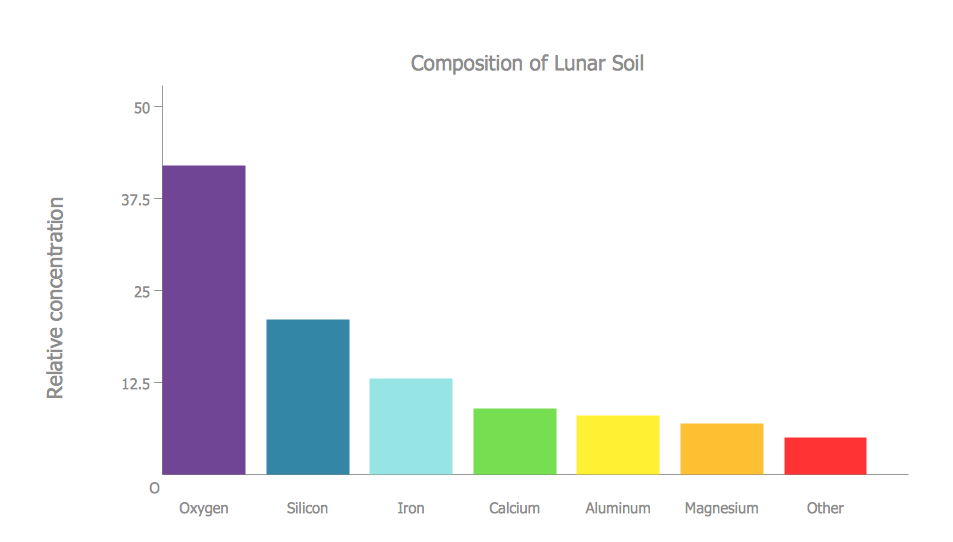## Sample 2: Manufacturing Productivity

Bar Graph sample: Manufacturing productivity.

This example is created using ConceptDraw DIAGRAM diagramming software enhanced with Bar Graphs solution from ConceptDraw Solution Park.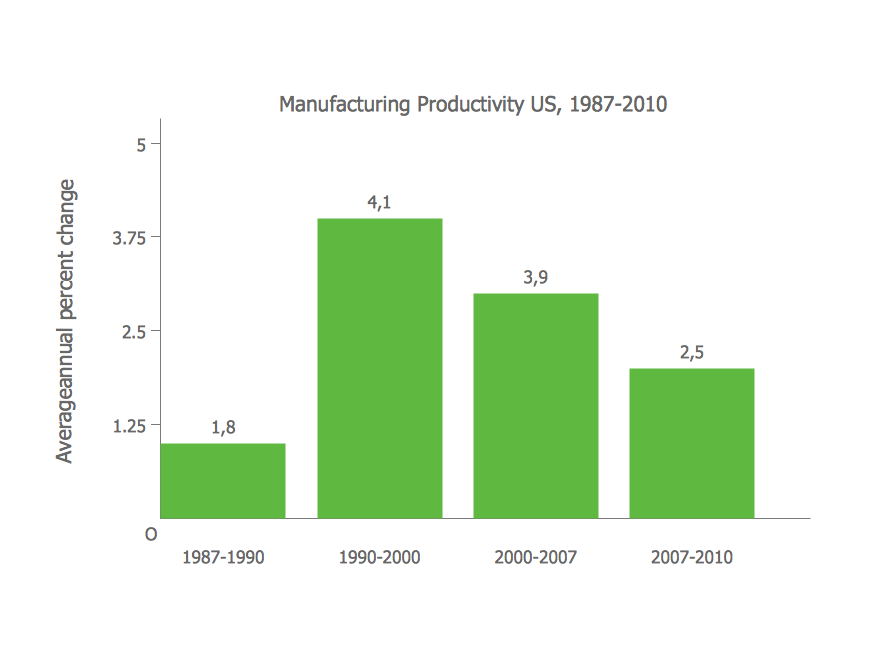## Sample 3: Average Rainfall

Bar Graph sample: Average rainfall.

This example is created using ConceptDraw DIAGRAM diagramming software enhanced with Bar Graphs solution from ConceptDraw Solution Park.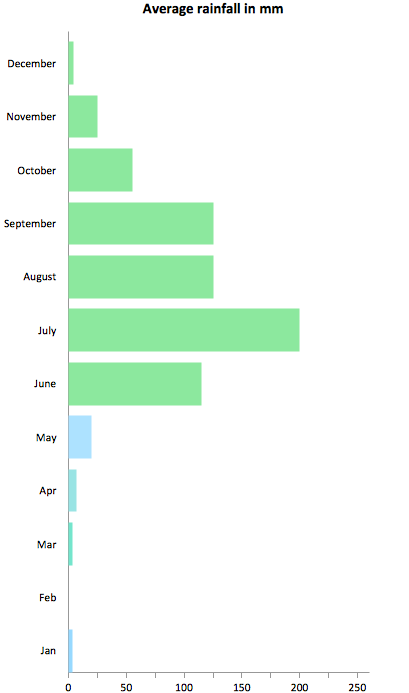## Sample 4: Survey on Why People Travel

Bar Graph sample: Survey on why people travel.

This example is created using ConceptDraw DIAGRAM diagramming software enhanced with Bar Graphs solution from ConceptDraw Solution Park.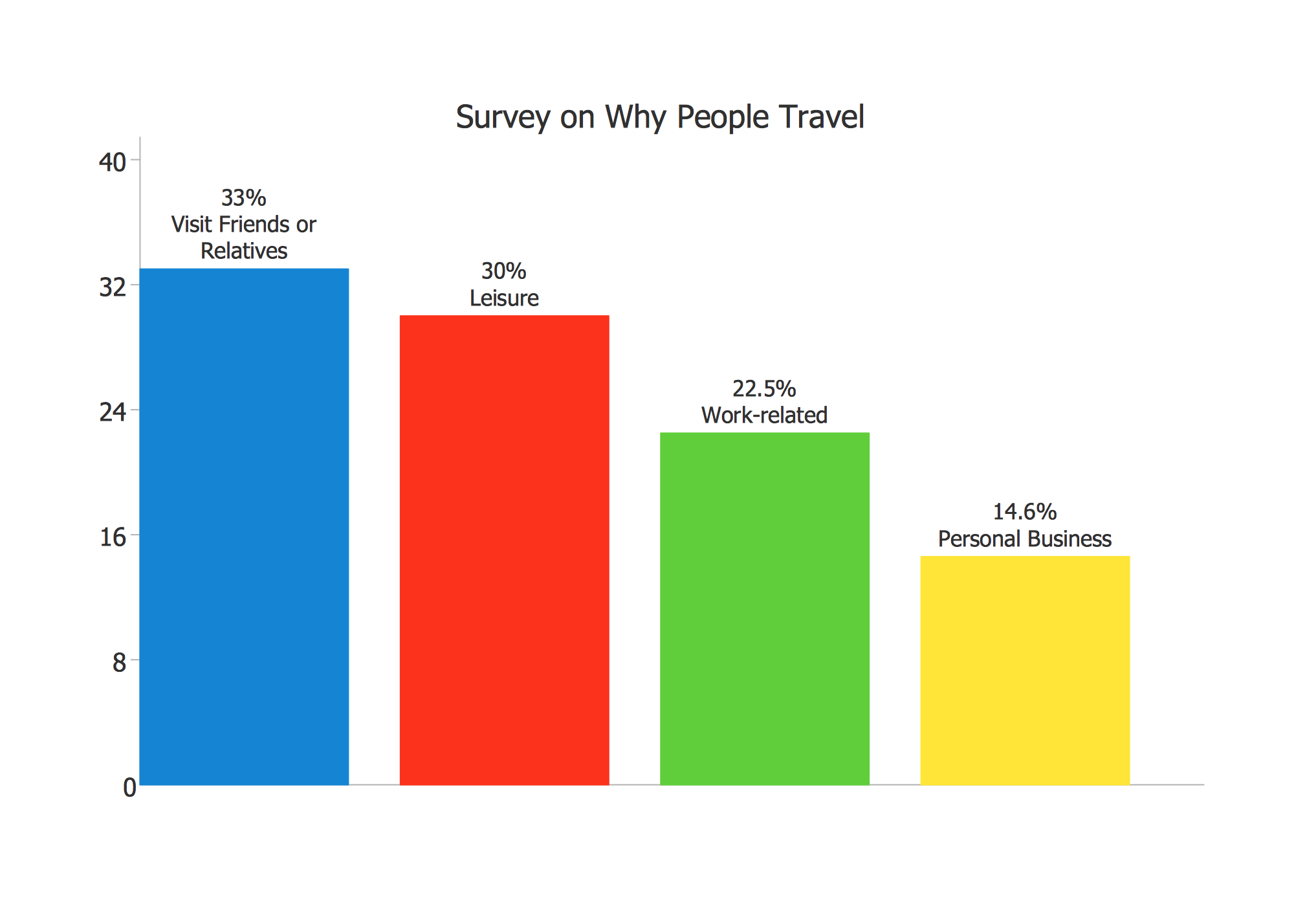## Sample 5: Times to Productivity

Bar Graph sample: Times to productivity.

This example is created using ConceptDraw DIAGRAM diagramming software enhanced with Bar Graphs solution from ConceptDraw Solution Park.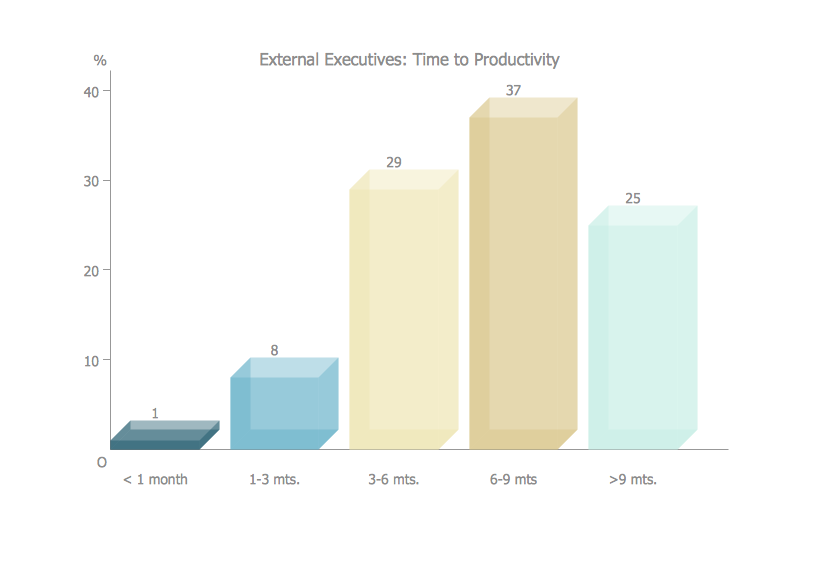## Sample 6: Waterfall

Bar Graph sample: Waterfall.

This example is created using ConceptDraw DIAGRAM diagramming software enhanced with Bar Graphs solution from ConceptDraw Solution Park.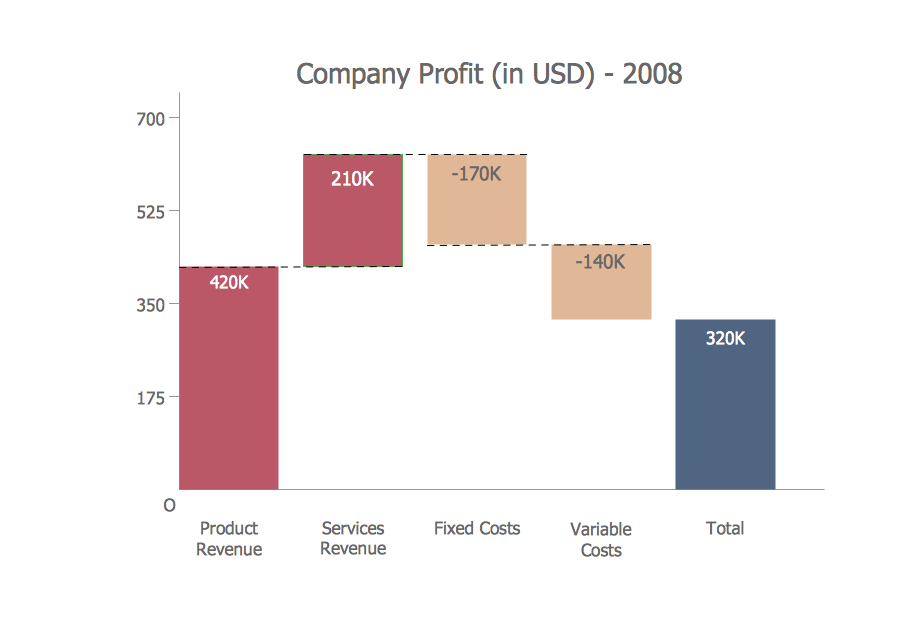## Sample 7: MIDEM-2011 Attendance by Region of the World

Bar Graph sample: MIDEM-2011 attendance by region of the world.

This example is created using ConceptDraw DIAGRAM diagramming software enhanced with Bar Graphs solution from ConceptDraw Solution Park.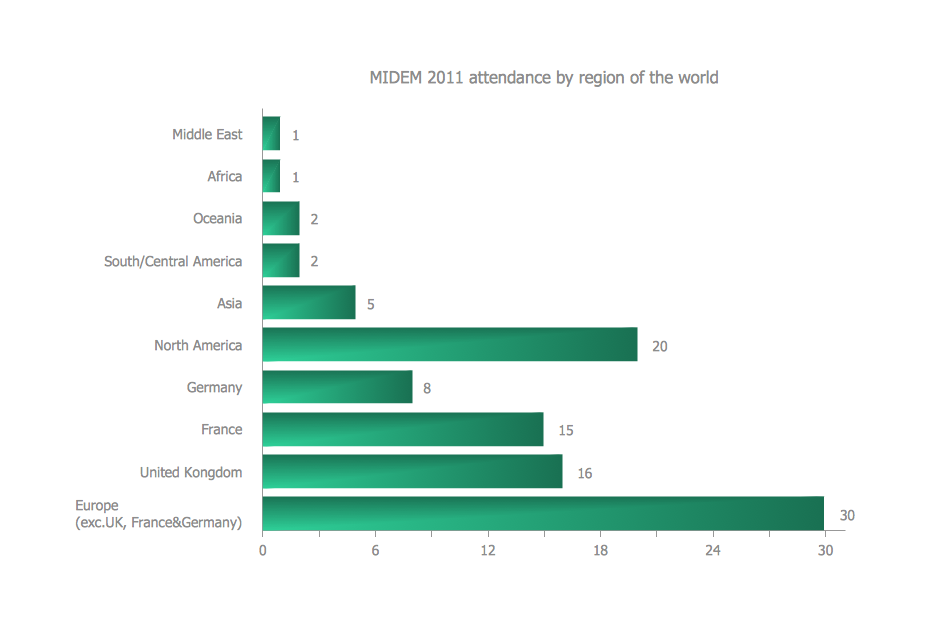## Sample 8: Changes in Price Indices

Bar Graph sample: Changes in price indices.

This example is created using ConceptDraw DIAGRAM diagramming software enhanced with Bar Graphs solution from ConceptDraw Solution Park.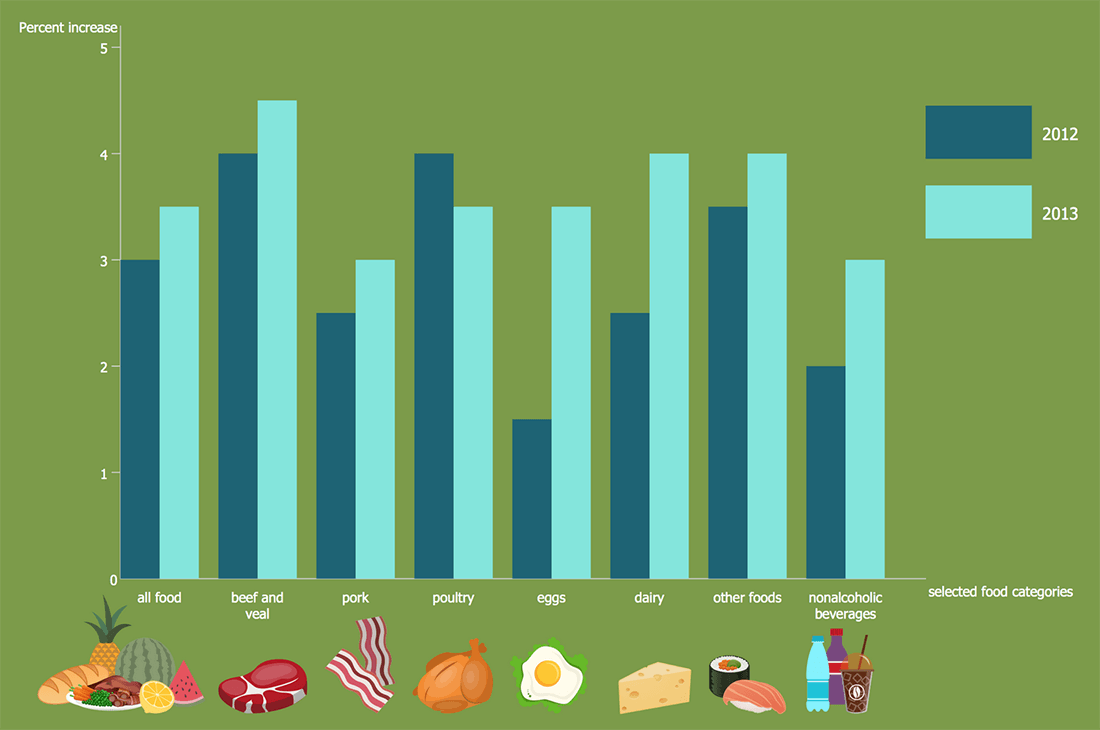## Sample 9: Global Competitiveness Index Infrastructure Score

Bar Graph sample: Global competitiveness index infrastructure score.

This example is created using ConceptDraw DIAGRAM diagramming software enhanced with Bar Graphs solution from ConceptDraw Solution Park.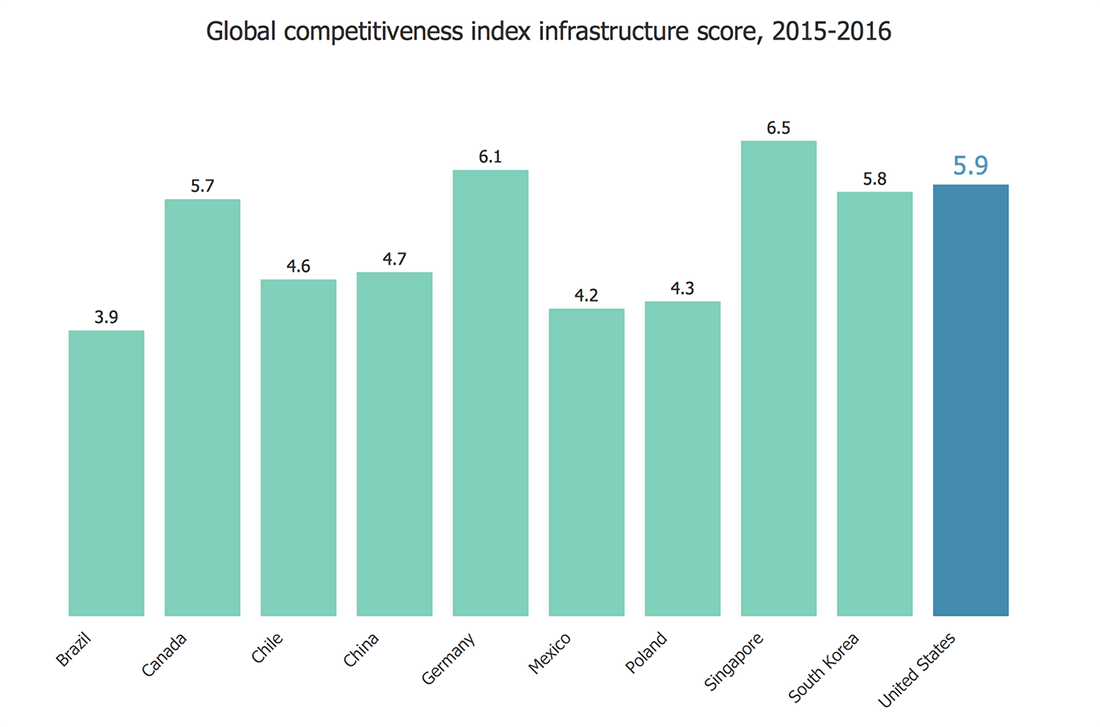## Sample 10: The Most Spoken Languages of the World

Bar Graph sample: The most spoken languages of the world.

This example is created using ConceptDraw DIAGRAM diagramming software enhanced with Bar Graphs solution from ConceptDraw Solution Park.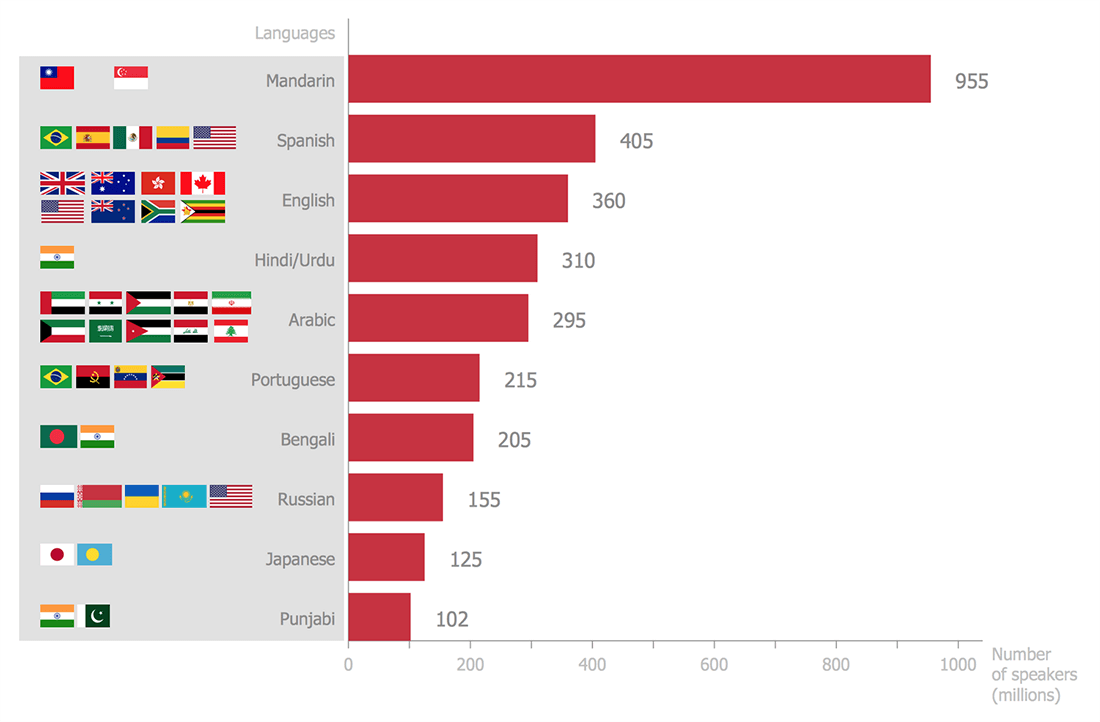## Sample 11: Average Trip Length Among U.S. Children

Bar Graph sample: Average trip length among U.S. children.

This example is created using ConceptDraw DIAGRAM diagramming software enhanced with Bar Graphs solution from ConceptDraw Solution Park.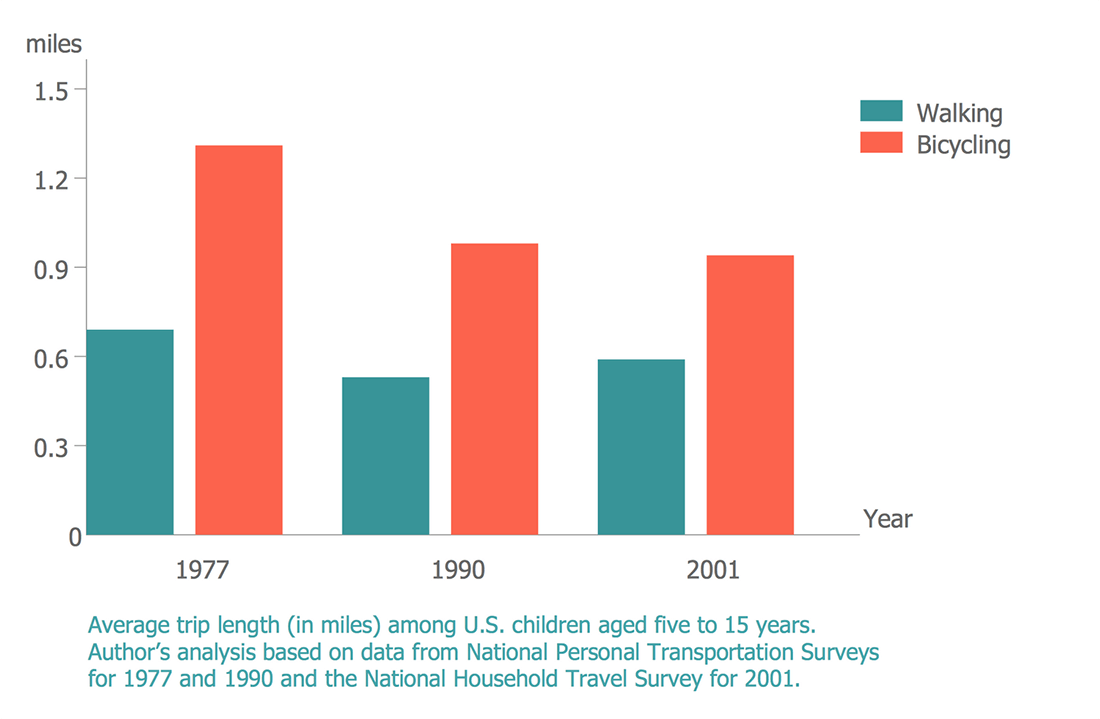## Sample 12: EPIET Fellows by Country of Origin

Bar Graph sample: EPIET fellows by country of origin.

This example is created using ConceptDraw DIAGRAM diagramming software enhanced with Bar Graphs solution from ConceptDraw Solution Park.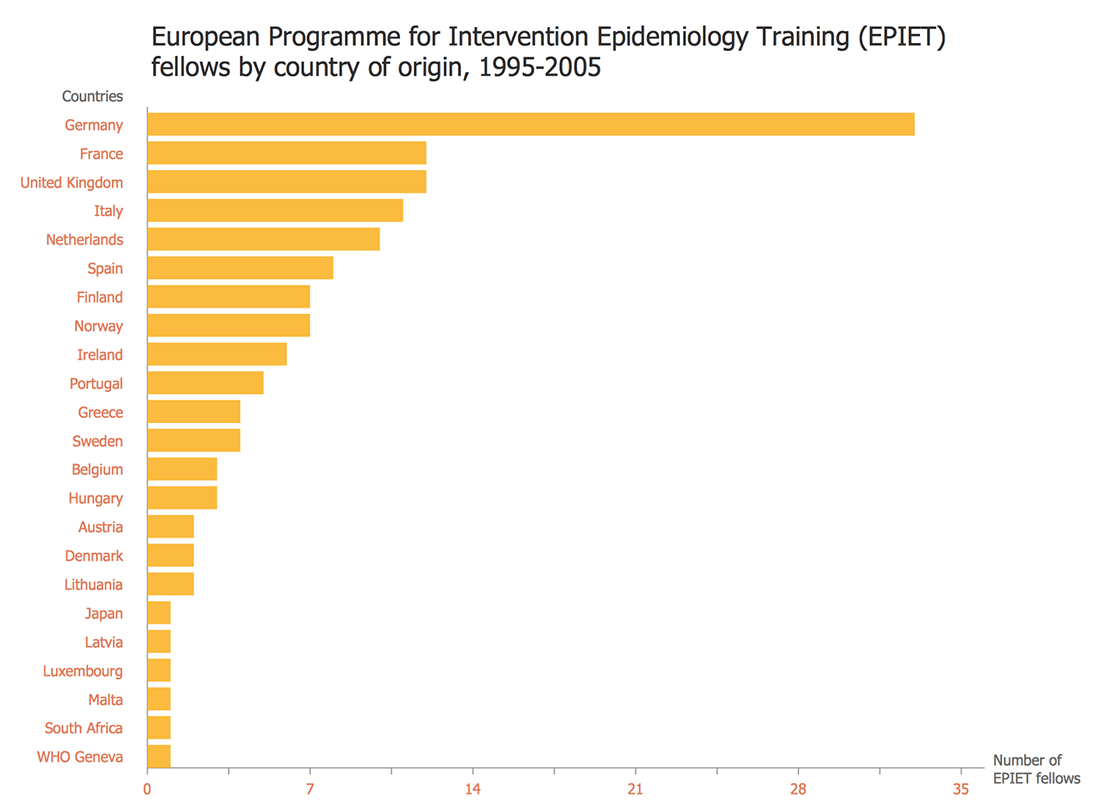## Sample 13: Amino Acid Composition of Complete UniProt Database

Bar Graph sample: Amino acid composition of complete UniProt database.

This example is created using ConceptDraw DIAGRAM diagramming software enhanced with Bar Graphs solution from ConceptDraw Solution Park.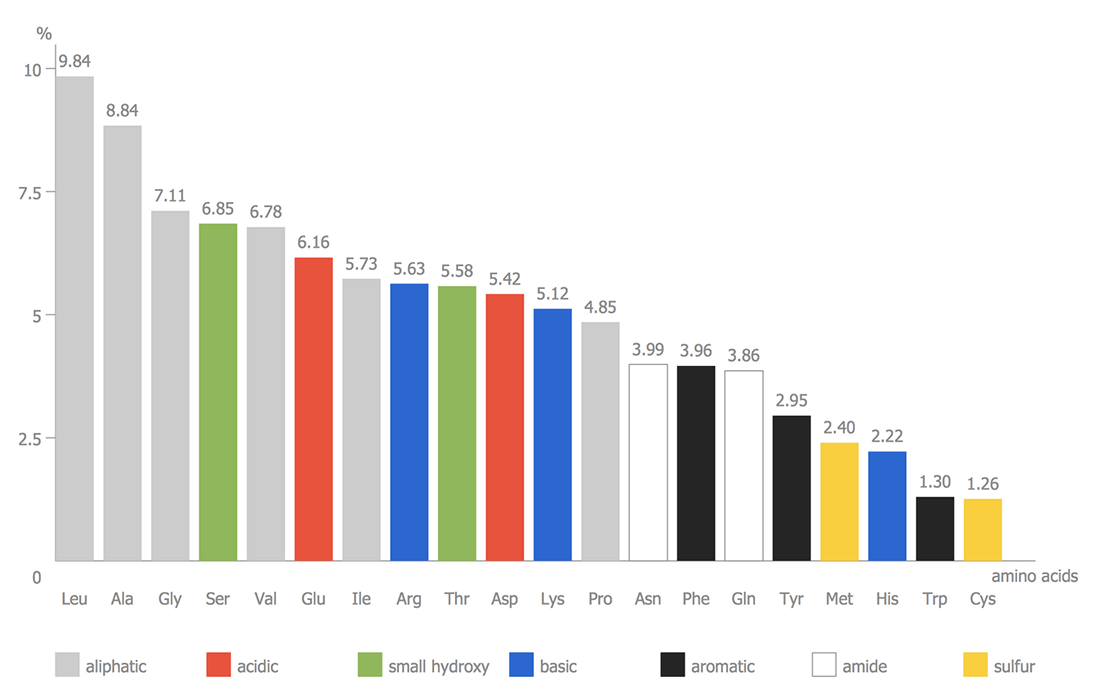## Sample 14: Iran Internet Users per 100 Person

Bar Graph sample: Iran internet users per 100 person.

This example is created using ConceptDraw DIAGRAM diagramming software enhanced with Bar Graphs solution from ConceptDraw Solution Park.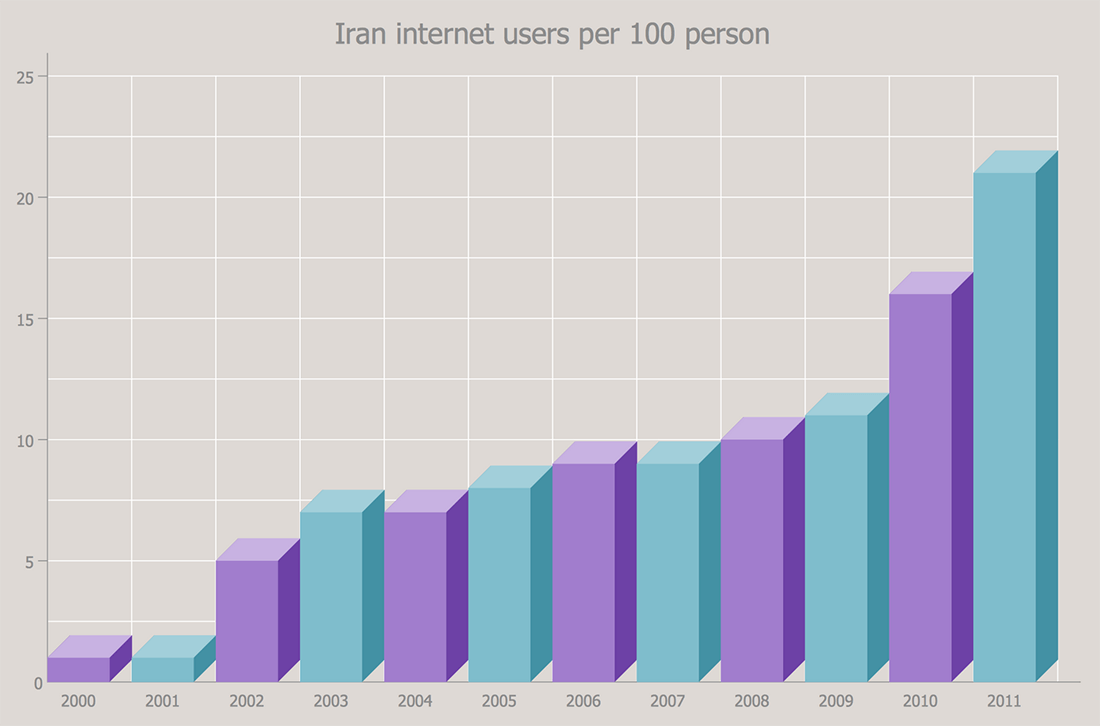## Sample 15: Median Income by Age and Marital Status

Bar Graph sample: Median income by age and marital status.

This example is created using ConceptDraw DIAGRAM diagramming software enhanced with Bar Graphs solution from ConceptDraw Solution Park.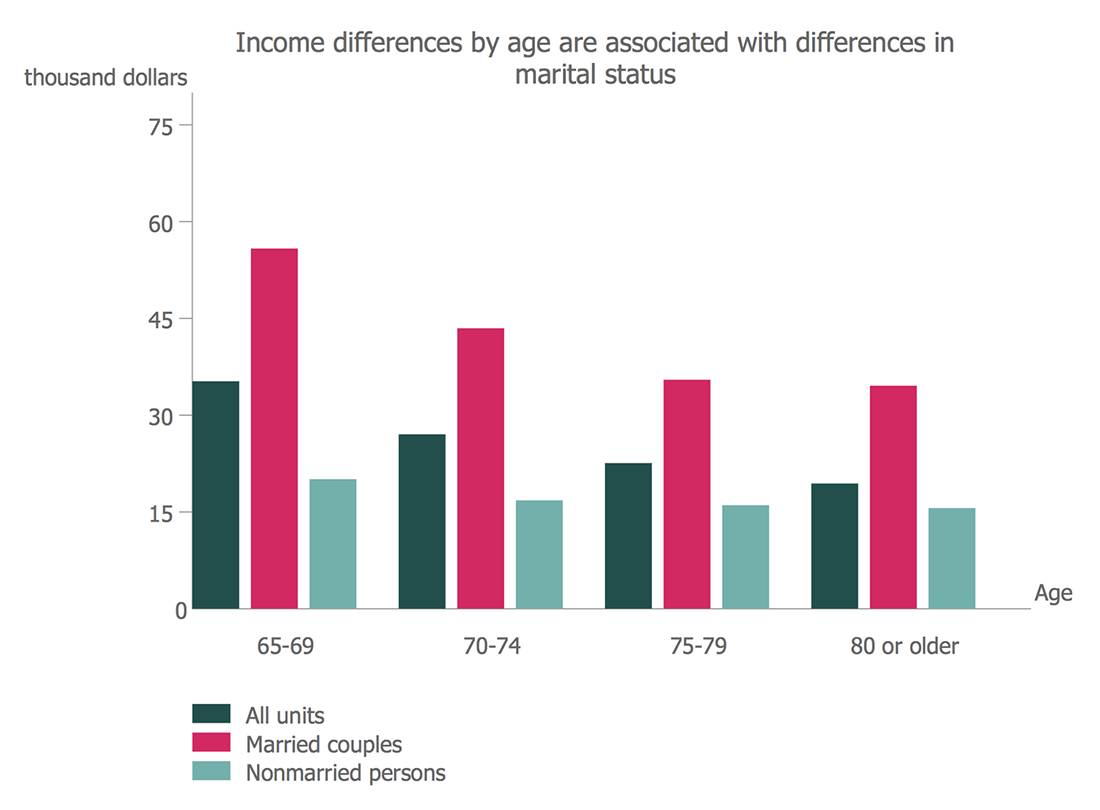## Sample 16: Nitrate Concentration in Community Water Systems (CWS)

Bar Graph sample: Nitrate concentration in Community Water Systems (CWS).

This example is created using ConceptDraw DIAGRAM diagramming software enhanced with Bar Graphs solution from ConceptDraw Solution Park.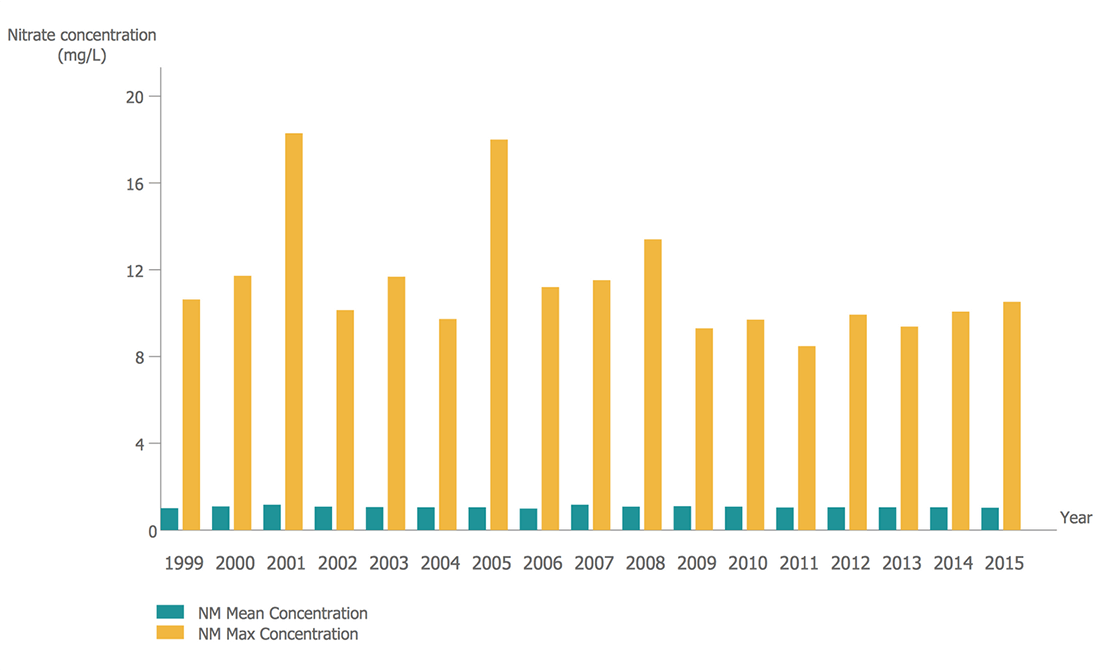## Sample 17: Number of Members in the CNT between 1911 and 1937

Bar Graph sample: Number of members in the CNT between 1911 and 1937.

This example is created using ConceptDraw DIAGRAM diagramming software enhanced with Bar Graphs solution from ConceptDraw Solution Park.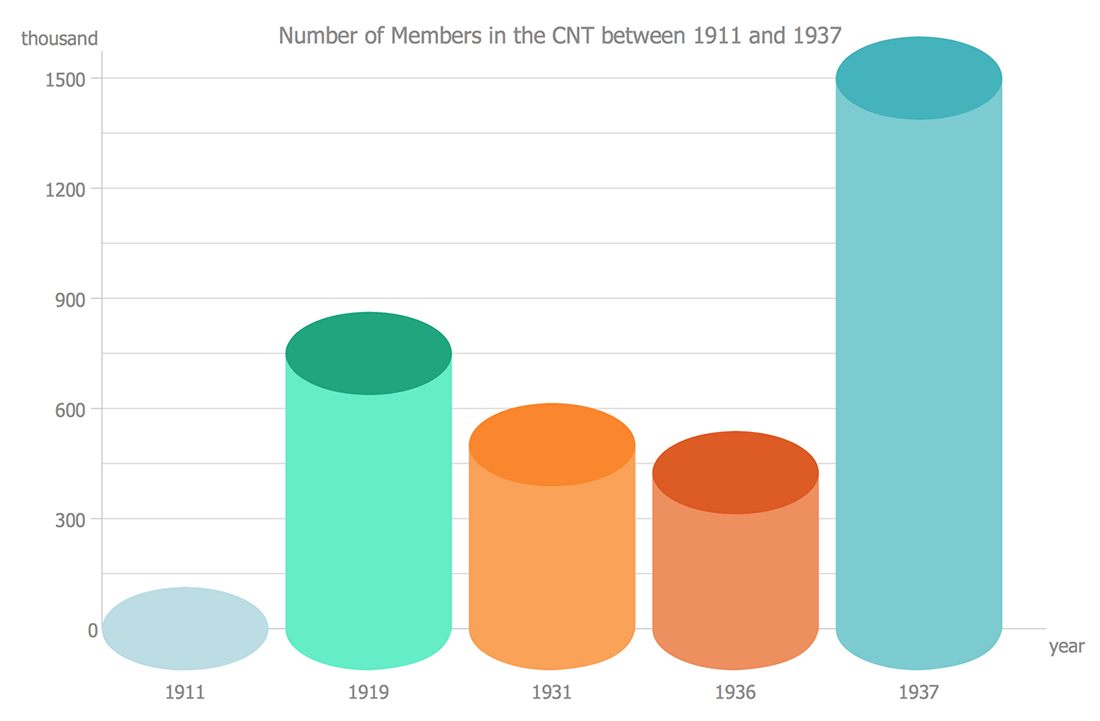## Sample 18: Percentage of U.S. High School Students

Bar Graph sample: Percentage of U.S. high school students.

This example is created using ConceptDraw DIAGRAM diagramming software enhanced with Bar Graphs solution from ConceptDraw Solution Park.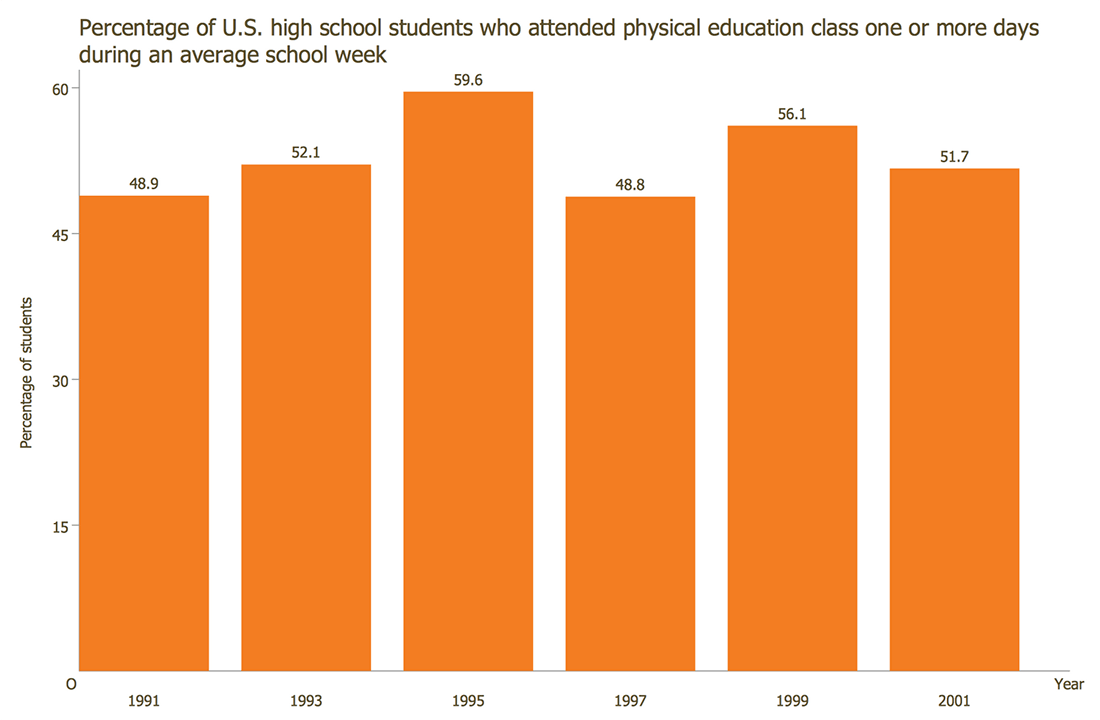## Sample 19: Percentage with Various Levels of Family Income

Bar Graph sample: Percentage with various levels of family income.

This example is created using ConceptDraw DIAGRAM diagramming software enhanced with Bar Graphs solution from ConceptDraw Solution Park.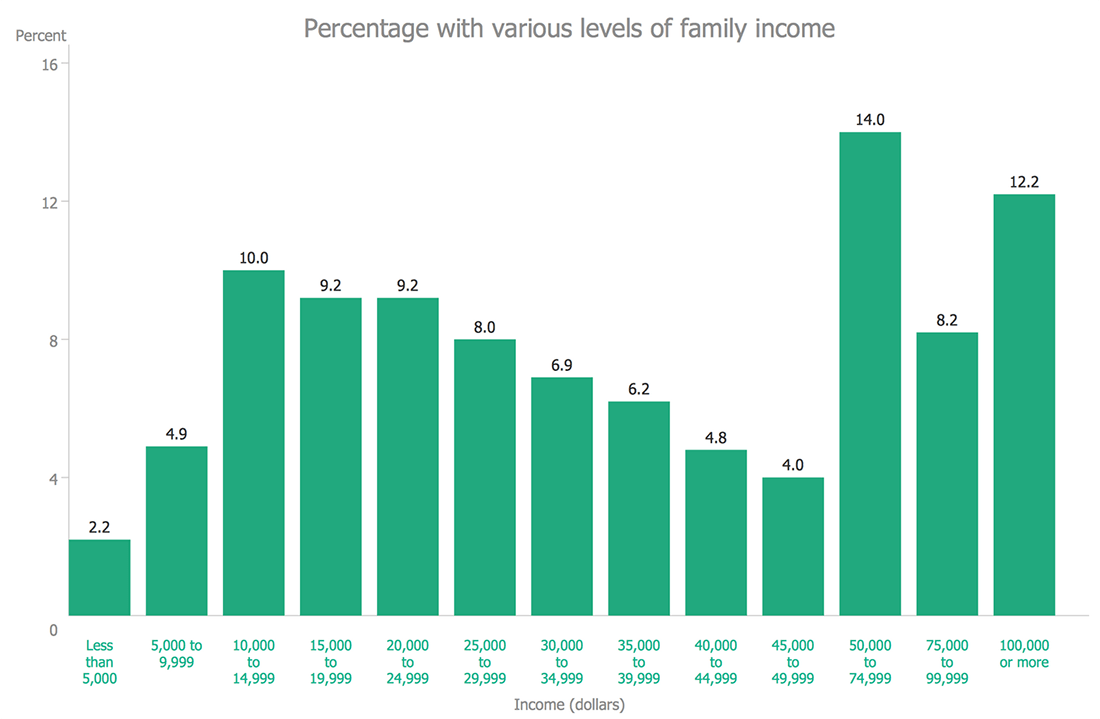## Sample 20: Serious Crime in Cincinnati

Bar Graph sample: Serious crime in Cincinnati.

This example is created using ConceptDraw DIAGRAM diagramming software enhanced with Bar Graphs solution from ConceptDraw Solution Park.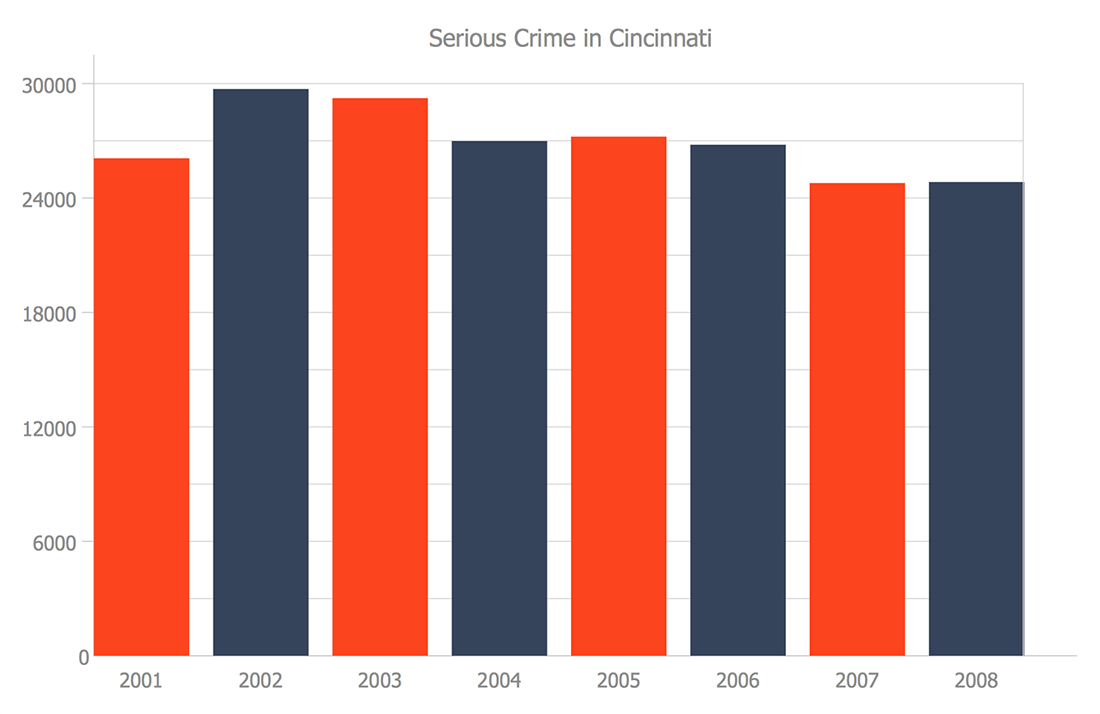All samples are copyrighted CS Odessa's.

Usage of them is covered by Creative Commons “Attribution Non-Commercial No Derivatives” License.

The text you can find at: https://creativecommons.org/licenses/by-nc-nd/3.0